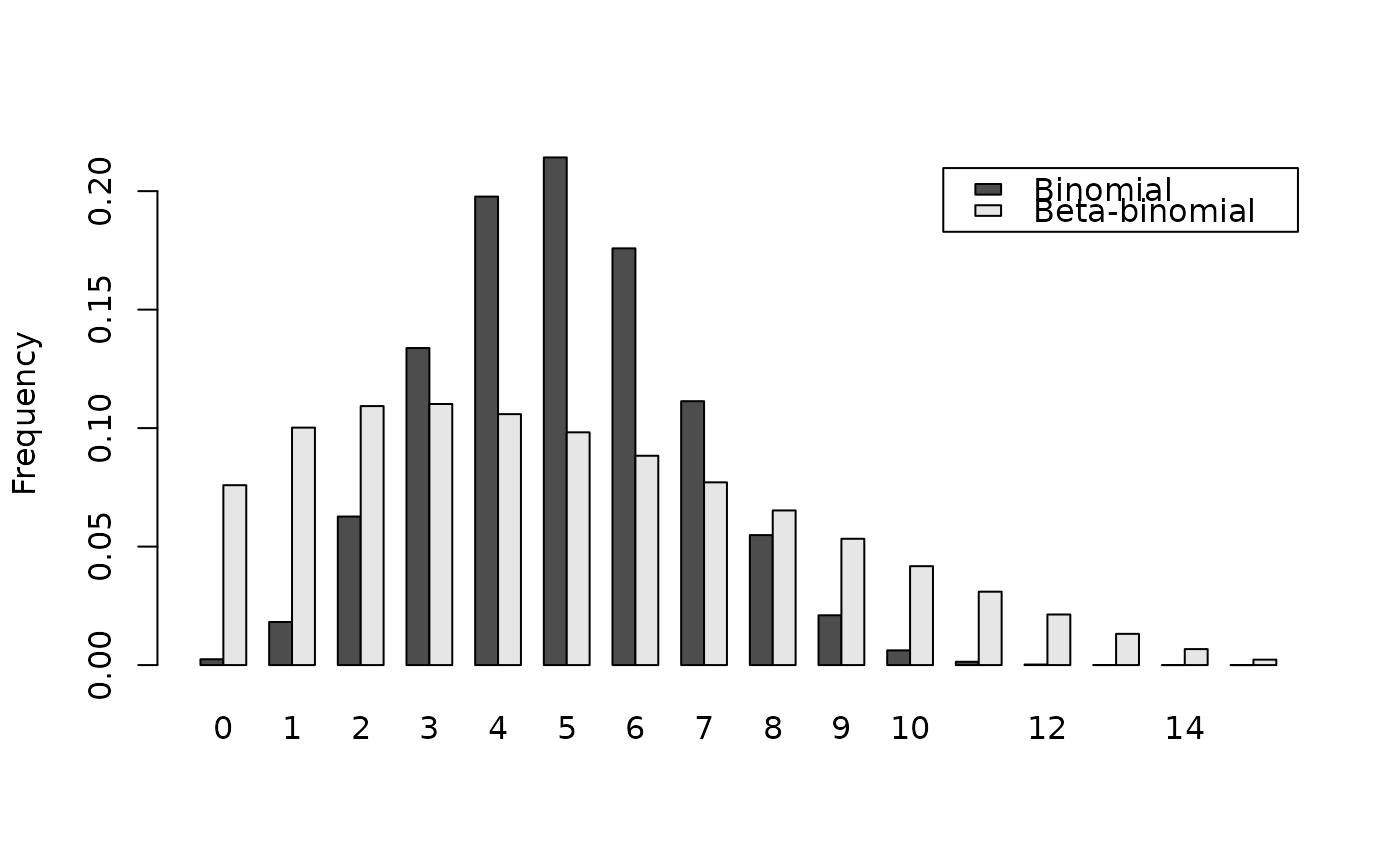Density, distribution function, quantile function and random generation for the beta-binomial distribution with parameters size, prob, theta, shape1, shape2. This distribution corresponds to an overdispersed binomial distribution.

dbetabinom(x, size, prob, theta, shape1, shape2, log = FALSE)

pbetabinom(q, size, prob, theta, shape1, shape2, lower.tail = TRUE,
log.p = FALSE)

qbetabinom(p, size, prob, theta, shape1, shape2, lower.tail = TRUE,
log.p = FALSE)

rbetabinom(n, size, prob, theta, shape1, shape2)

## Arguments

x, q Vector of quantiles. Number of trials. Probability of success on each trial. Aggregation parameter (theta = 1 / (shape1 + shape2)). Shape parameters. Logical; if TRUE, probabilities p are given as log(p). Logical; if TRUE (default), probabilities are $$P[X \le x]$$ otherwise, $$P[X > x]$$. Vector of probabilities. Number of observations.

## Value

dbetabinom gives the density, pbetabinom gives the distribution function, qbetabinom gives the quantile function and rbetabinom generates random deviates.

## Details

Be aware that in this implementation theta = 1 / (shape1 + shape2). prob and theta, or shape1 and shape2 must be specified. if theta = 0, use *binom family instead.

dbetabinom in the package emdbook where the definition of theta is different.

## Examples

# Compute P(25 < X < 50) for X following the Beta-Binomial distribution
# with parameters size = 100, prob = 0.5 and theta = 0.35:
sum(dbetabinom(25:50, size = 100, prob = 0.5, theta = 0.35))#>  0.3054911
# When theta tends to 0, dbetabinom outputs tends to dbinom outputs:
sum(dbetabinom(25:50, size = 100, prob = 0.5, theta = 1e-7))#>  0.5397943sum(dbetabinom(25:50, size = 100, shape1 = 1e7, shape2 = 1e7))#>  0.5397944sum(dbinom(25:50, size = 100, prob = 0.5))#>  0.5397945
# Example of binomial and beta-binomial frequency distributions:
n   <- 15
q   <- 0:n
p1  <- dbinom(q, size = n, prob = 0.33)
p2  <- dbetabinom(q, size = n, prob = 0.33, theta = 0.22)
res <- rbind(p1, p2)
dimnames(res) <- list(c("Binomial", "Beta-binomial"), q)
barplot(res, beside = TRUE, legend.text = TRUE, ylab = "Frequency")# Effect of the aggregation parameter theta on probability density:
thetas <- seq(0.001, 2.5, by = 0.001)
density1 <- rep(sum(dbinom(25:50, size = 100, prob = 0.5)), length(thetas))
density2 <- sapply(thetas, function(theta) {
sum(dbetabinom(25:50, size = 100, prob = 0.5, theta = theta))
})
plot(thetas, density2, type = "l",
xlab = expression("Aggregation parameter ("*theta*")"),
ylab = "Probability density between 25 and 50 (size = 100)")lines(thetas, density1, lty = 2)